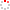#CodeStepByStep## wordStatsPlus

Language/Type: C++ basics streams file input

Write a function named `wordStatsPlus` that accepts as its parameter a string holding a file name, opens that file and reads its contents as a sequence of words, and produces a particular group of statistics about the input. You should report: the total number of lines; total number of words; the number of unique letters used from A-Z, case-insensitively, and its percentage of the 26-letter alphabet; the average number of words per line (as an un-rounded real number); and the average word length (also un-rounded). For example, suppose the file `tobe.txt` contains the following text:

```To be  or not  TO BE,
THAT  IS

really   the  question.
```

For the purposes of this problem, we will use whitespace to separate words. That means that some words include punctuation, as in "be,". For the input above, the call of `wordStatsPlus("tobe.txt");` should produce exactly the following output. The number of "unique letters" is 14 because the file contains 14 distinct letters of the alphabet from A-Z: a, b, e, h, i, l, n, o, q, r, s, t, u, and y.

```Total lines = 4
Total words = 11
Total unique letters = 14 (53% of alphabet)
Average words/line = 2.75
Average word length = 3.45455
```

If the input file does not exist or is not readable, your function should instead print the following output:

```Error, bad input file.
```

Constraints: Your solution should read the file only once, not make multiple passes over the file data.

Type your C++ solution code here:

This is a function exercise. Write a C++ function as described. Do not write a complete program; just the function(s) above.